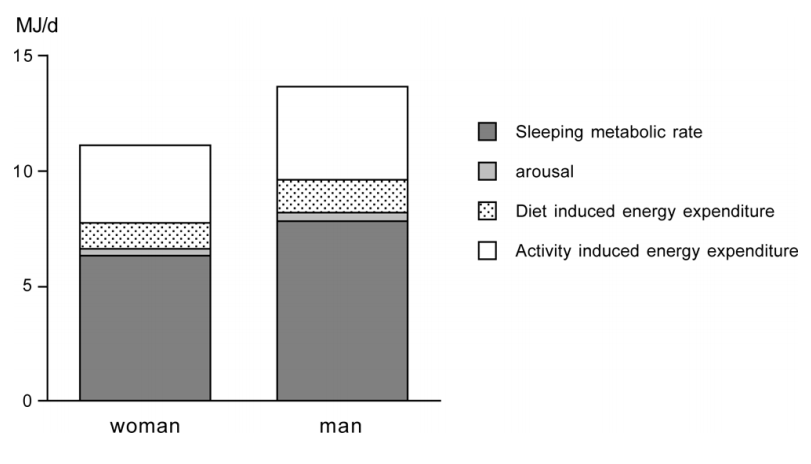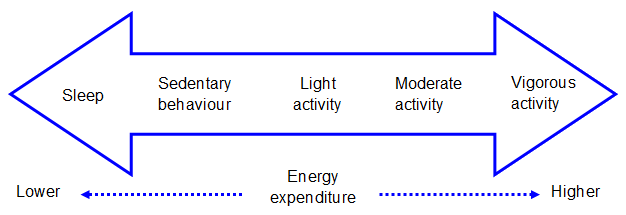#### Energy expenditure

Contents

Human consumption and expenditure of energy, alongside any change in the body’s macronutrient stores (fat, protein, and carbohydrate) is summarised by the energy balance equation:

Change in macronutrient stores = Energy consumed – Energy expended
• The individual parts of the equation can be expressed as kilojoules (kJ) or as kilocalories (kcal); 1 kcal is equivalent to 4.2 kJ.
• The SI unit of energy is Joules which is therefore generally preferred; however kcal is also commonly used as this unit of energy is defined by the concept of heat.
• The two behavioural components, energy intake and energy expenditure, are usually expressed per unit of time, for example Megajoules per day

As depicted by Figure P.1.1, total human energy expenditure consists of the following elements:

• Sleeping metabolic rate
• The energy cost of wakefulness (also termed arousal)
• Physical activity energy expenditure (PAEE), the energy above rest expended during body movement and postures
• Diet-induced energy expenditure (also known as the thermic effect of food), which is associated with digestion, absorption and storage of food

Sleeping metabolic rate and the energy cost of arousal together make up resting energy expenditure. This is similar to basal energy expenditure or basal metabolic rate, although the latter has a more strict definition of how it is measured; in the fasted state at complete rest the morning after sleep. This is the energy required to maintain cellular function. Resting metabolic rate is slightly higher than basal metabolic energy expenditure (typically within 10%).

Resting energy expenditure is normally the largest component of daily energy expenditure, and is greater in individuals of greater mass, particularly fat-free mass. Physical activity energy expenditure is the most variable day-to-day and is directly related to movement. Due to their close relationship with body size, these components are typically adjusted for body mass or fat-free mass.Figure P.1.1 Components of total energy expenditure for an average young adult woman and man. Source: Westerterp, 2013.

• Physical activity can be defined as any bodily movement produced by skeletal muscles resulting in energy expenditure.
• Physical activity energy expenditure depends on the amount of body movement and the size of the body, since it takes more energy to move more mass
• Total physical activity energy expenditure for a defined time-frame (be it an hour, day, week etc.) is the product of the intensity, duration and frequency of body movement
• The rate of physical activity energy expenditure per unit time is commonly referred to as activity intensity
• The rate of energy expenditure or intensity spectrum (see Figure P.1.2) ranges from behaviours with very low energy expenditure, such as sleep, to vigorous activities with high energy expenditure such as sprinting
• Physical activity is the broad label that encompasses all movement of at least light intensity, and extends to movement of moderate and vigorous intensityFigure P.1.2 The continuum of human movement and energy expenditure. Source: BHFNC for Physical Activity and Health, 2012.

• The intensity of physical activity can be expressed relative to an individual’s resting energy expenditure, referred to as a metabolic equivalent of task (MET)
• Individual activities are assigned a MET value representing their energy cost relative to this resting value
• For example, a MET value of 2.0 represents a doubling of the resting rate of energy expenditure and would be indicative of light physical activity
• A compendium of behaviours and their associated MET scores was originally published in 1993 and updated in 2011 to include 821 activities ranging from sleep (0.9 MET) to running at 14.0 mph (23.0 METs)